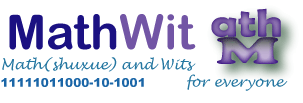# Welcome to MathWit!

We provide some vaulable Math and Personal Finance Resources and offer one to one online math tutorials from high school to advanced university levels. Sample subjects:

• Math IB HL(or SL but HL preferred)
• AP Calculus BC
• Basic computer programming and math, statistics software
• Introduction to math contest and math writing using latex
• Basic computer programming and math, statistics software
• R
• Mathematica
• Python
• SPSS
• Rust ......